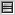Table of Contents
1. Programming Language Comparison - Jonathan Pryor's web log
1. Programming Language Comparison

# Programming Language Comparison - Jonathan Pryor's web log

## Programming Language Comparison

Last year, Joel Spolsky mentioned the The Perils of JavaSchools, and mentioned the mid-term he took at Penn:

1a. (MIT-Scheme) Using the following function, implement sum-of-squares which calculates the sum of squares of a list:

```(define (accumulate combiner null-value l)
(if (null? l)
null-value
(combiner (car l)
(accumulate combiner
null-value
(cdr l)))))

(define (sum-of-squares l)
(accumulate (lambda (x y) (+ (* x x) y))
0
l))

(sum-of-squares '(1 2 3 4 5))
```

Simple, straightforward (if you're familiar with the functional programming paradigm), and 14 lines of code.

This begs the question: what's the similar flavor code in C# and Java?

C# 2.0 allows anonymous delegates, which allows an almost direct conversion of the Scheme version, with the caveats that this version is limited to ints (while Scheme will handle mixed numeric types), and it's a more verbose 25 lines, largely because of the required class structure:

```// Sum Of Squares
using System;
using System.Collections;
class Exam {
delegate int Combiner (int a, int b);

static int Accumulate (Combiner combiner, int null_value, IEnumerator l)
{
if (!l.MoveNext())
return null_value;
return combiner ((int) l.Current,
Accumulate (combiner, null_value, l));
}

static int SumOfSquares (IEnumerable e)
{
return Accumulate (
delegate (int a, int b) { return a*a + b; },
0, e.GetEnumerator ());
}

public static void Main ()
{
Console.WriteLine (SumOfSquares (new int[]{1, 2, 3, 4, 5}));
}
}
```

Notice that I'm using IEnumerator.Current as the (car) of the list and the IEnumerator instance is the (cdr) of the list. This is a reasonable mapping, and allows any .NET collection type to be used with the Exam.SumOfSquares method.

Java is considerably more verbose to stay in the same style as the Scheme program, requiring 37 lines. It's similar to C# in that it uses the standard Iterator interface to represent a list, with next() mapping to (car) and the Iterator instance containing (cdr). This program also uses an interface declaration and an anonymous inner class to implement the callback (to maintain the feel from the Scheme program):

```import java.util.*;

class sos {
static interface Combiner {
int combine (int a, int b);
}

static int accumulate (Combiner combiner, int null_value, Iterator l) {
if (!l.hasNext())
return  null_value;
Object next = l.next ();
return combiner.combine (
((Integer) next).intValue (),
accumulate (combiner, null_value, l));
}

static int sumOfSquares (Iterator l) {
return accumulate (
new Combiner () {
public int combine (int a, int b) {
return a*a + b;
}
},
0, l
);
}

public static void main (String[] args) {
List l = new ArrayList ();